# Every single year, exam boards create a public document written by the people who mark exam papers.

In the document, examiners tell you what the common mistakes were, where student’s missed marks, examples of what to do and past topics for that particular past paper. This is different to a past paper mark scheme as it gives you the examiners insights rather than the correct answer.

These are incredibly useful resources which give you the most valid and up-to-date information, straight from the people who decide on awarding the mark. You should use the examiner’s report as much as you possibly can! Print it off and stick it above your desk or next to your bed. Knowing what examiners have consistently awarded marks for in the past 5 years can make the difference between an A and A* – do not underestimate the power of knowledge!

In this post we will dissect the May 2017 AQA Chemistry past paper (the most recent available as of 01/04/19).

### General Statements by AQA Examiners for the Chemistry A-level past paper

The paper showered a perfect bell curve with a mean score of 45.7%. The answers were all deemed “achievable”, but some questions demanded a unique approach than in previous papers. The most difficult questions in this paper were 3.3 and 4.2. A key theme noted was that students did not take the time to properly read the question carefully – make sure you do not lose marks like this!

### “ER” in this post stands for EXAMINERS REPORT/REMARKS

 Q1.1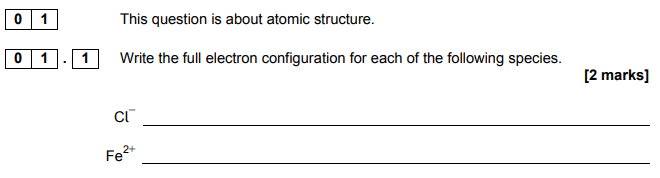ER & ANSWER A well answered question but many students were unable to write the electronic configuration for Fe2+ so make sure you cover this topic.Q1.2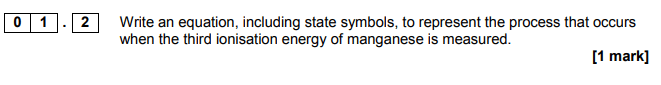ER Generally well answered but many students didn’t include state symbols which are explicitly asked for in the question. Make sure you read the question thoroughly.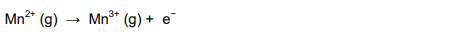Q1.3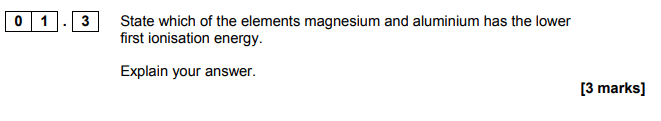ER A familiar question to many students. This meant easy marks to be gained, as always brush up on the basics.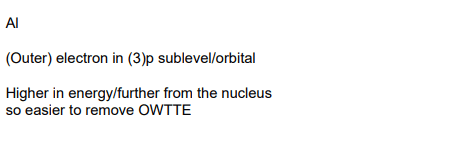Q1.4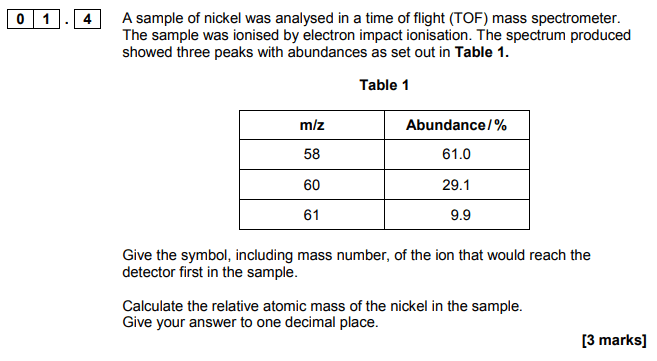ER The relative atomic mass was straightforward to many students got this correct, but the symbol including the mass number was often incorrect. Again, make sure you read the question properly!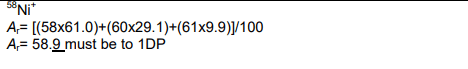Q2.1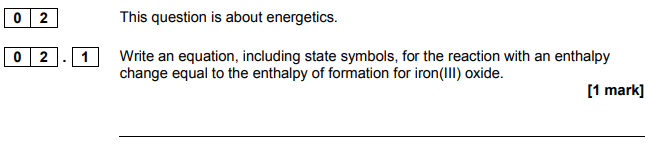ER A low mark question but very poorly answered. Only 26.4% of students got the mark here as most missed state symbols and balanced the equation incorrectly. Balancing equations comes up very frequently in this paper and most Chemistry A-level papers. Make sure you’re confident with balancing equations.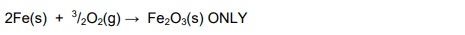Q2.2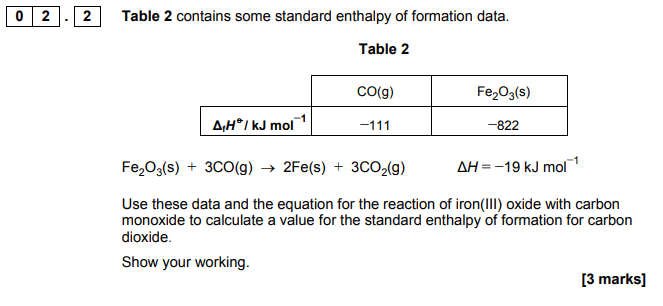ER This question was considerably harder than previous paper’s questions on the same topic. 34.3% of students didn’t even get 1 mark. Always be wary of more challenging questions than you may have experienced before – try and challenge yourself when revising so rogue questions like this don’t catch you off-guard.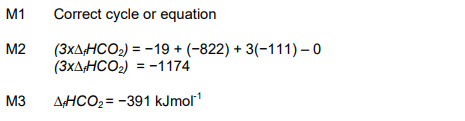Q2.3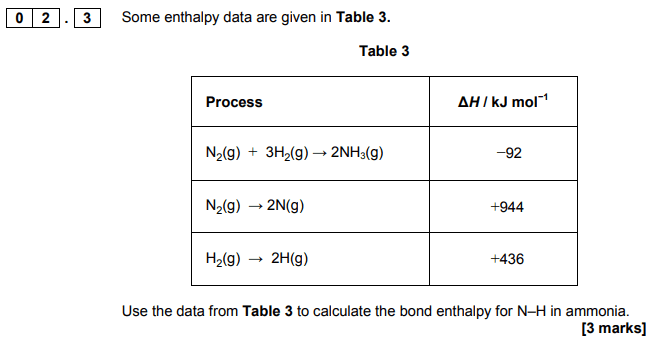ER Another very difficult question, only 26.3% got the mark available here.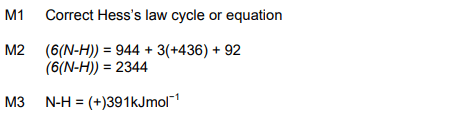Q2.4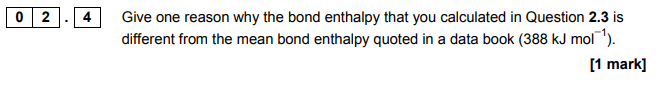ER This surprised examiners as they expected it to be better answered – “The fact that mean bond enthalpies are calculated using a range of compounds was not well understood.”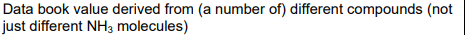Q3.1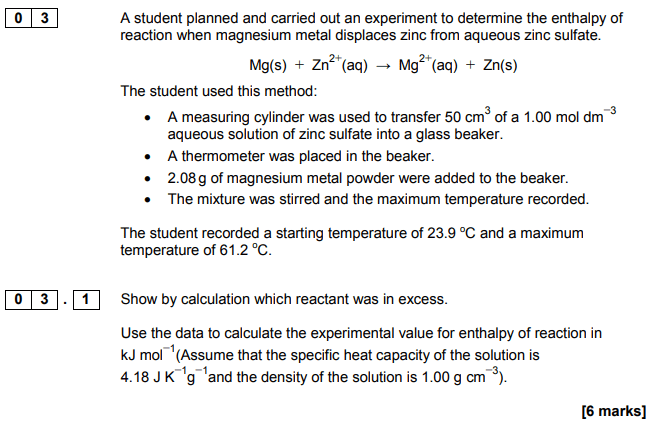ER To get full marks the correct limited reagent had to be used – this was challenging for many students. Less able students were expected to calculate the number of moles of the two reagents. There are often a few ways to get to an answer on higher marked questions. Explore all avenues before giving up.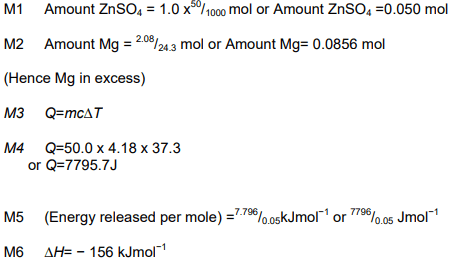Q3.2ER An easy question with an easy mark for 58.1% of students.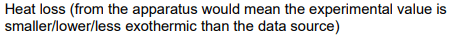Q3.3ER A very difficult question and worth a lot of marks (13.3% of the entire paper’s available marks). There were a wide variety of answers, highlighting to examiners that the practical had been carried out at their school. Again, many students failed to read the question carefully and didn’t clearly justify how the method could be improved. Don’t let this be you!Q4.1ER Only 36.3% of students gained a mark. This was a theme throughout with poor scores on algebraic questions. If you are poor on a particular topic, don’t neglect it in revision! It will be the thing that helps you gain the most marks.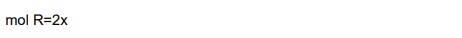Q4.2ER There was an “elegant” and easier approach for this question (taking the square root on both sides of the equation), but not many students identified this and thus, many missed out on full marks and made the question much harder for themselves. Keep a keen eye for shortcuts – if a question seems unnecessarily difficult, it might be there’s an easier route to the answer.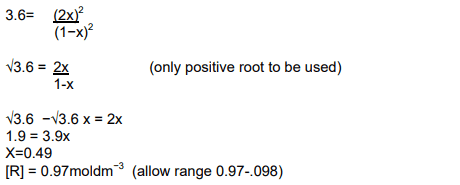Q5.1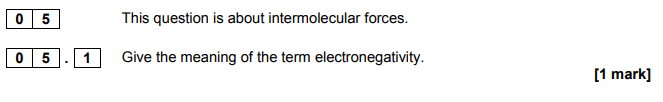ER 51.8% of students got this mark – a pretty straightforward question.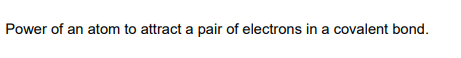Q5.2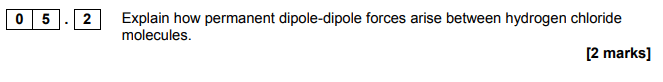ER The second mark in this question was very rarely scored, again pointing to students possibly not reading the question properly. “The best answers included a diagram.” Where possible, make answering the question faster and illustrate your answer!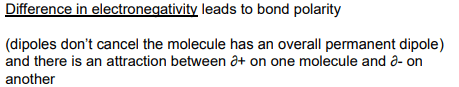Q5.3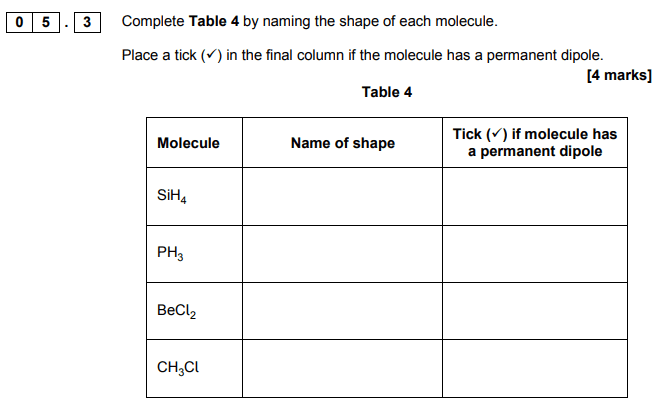ER The shapes were well known but many students didn’t get the link with polarity. When revision try and link all the topics you learn together in case there are links to be made between them.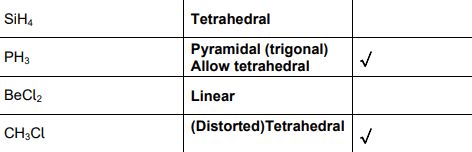Q6.1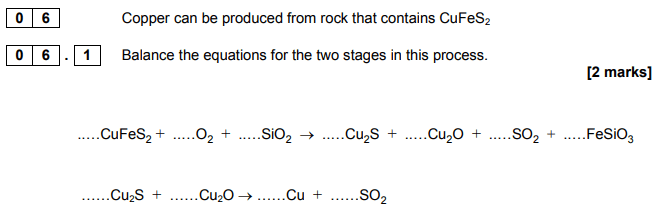ER Only 20.8% of students gained both marks here. Not many students got the first question but the second was answered much better. Again, make sure you can balance equations for Chemistry. Physics A-level may mean make sure you know Electricity very well etc.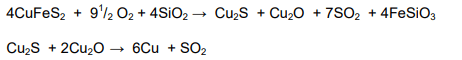Q6.2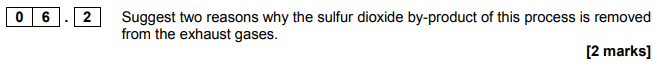ER Although the context was quite unfamiliar for this specification, 86.3% of students picked up at least one mark in the question.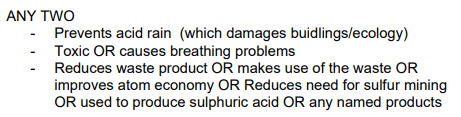Q6.3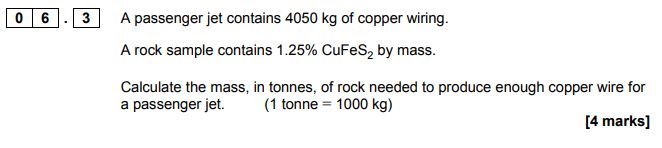ER A very difficult question with 53.3% of students not gaining a mark at all. Examiners put the difficult of this question down to the context and changes of units. Try not to let the context of a question throw you off – if it’s in the paper, you will have covered it at some point.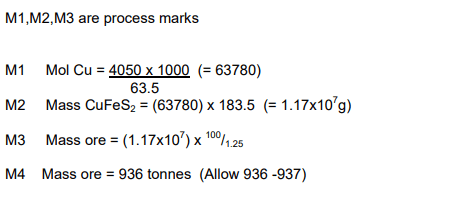Q6.4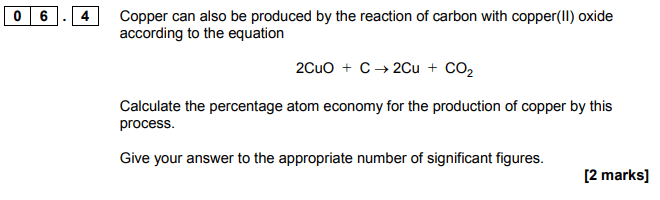ER Candidates scored much better on this question due to the familiarity of the calculation compared to the previous question.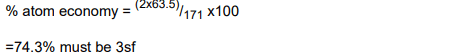Q7.1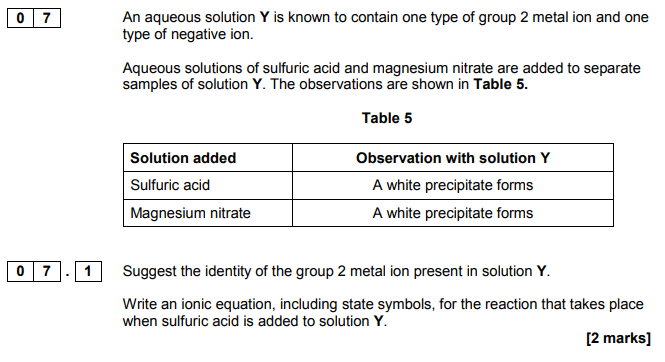ER The ions were identified correctly very often but the equation was much less well answered. Difficult equations have been a theme throughout the paper.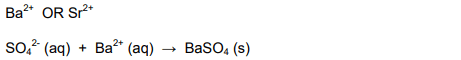Q7.2ER “Even good students struggled with this question.” The context of the ion test was reversed so the question was poorly understood by most students. This is a common tactic employed by exam boards to frame questions in a different light.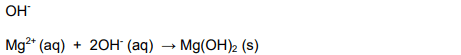Q8.1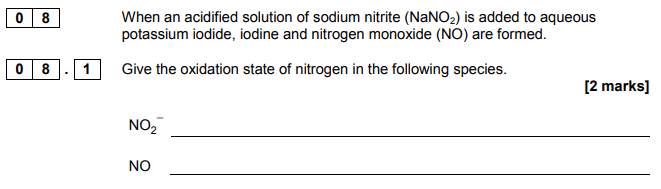ER A very well answered question with 71.8% of students gaining both marks on this. This highlights the benefit of studying the basics too.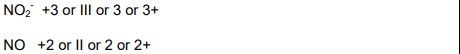Q8.2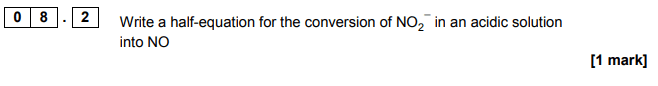ER This surprised AQA examiners because in 2016 most students didn’t get the mark. This year, 44.6% of students gained the mark.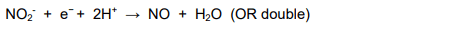Q8.3ER 62.4% of students gained the mark for this half-equation – more in line with what examiners were expecting.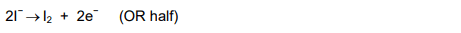Q8.4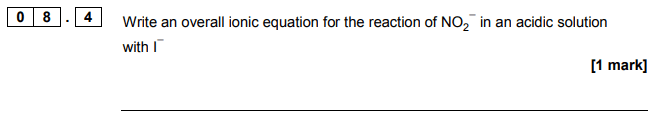ER A massive 8.5% of students didn’t even attempt this question – when there’s no negative marking and you have time you should always attempt the question.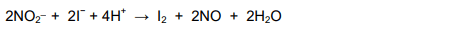Q8.5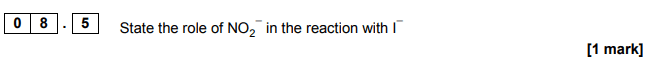ER 56.3% of students gained the mark – another easy mark if you brushed up on the basics.Q8.6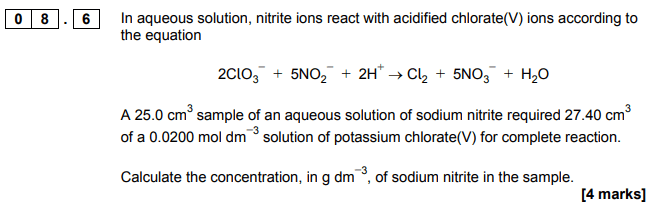ER Despite the challenging mole ratio, this question was answered better than question 6.3. This was due to the context and framing of the question. Make sure you truly understand the question before attempting to answer it.## Multiple Choice Section B

The multiple choice section is generally easier and students gain more marks. This is because of a combination of logical reasoning (eliminating choices that can’t be correct) and guessing answers students don’t know at a 25% chance of success. In this section, the incorrect answers are named “distractors” – usually the answer gained from a common incorrect calculation or assumption.

As there are no multiple part questions in this section, we will simply state the question number and ER (Examiners report) on the same line.

 Q9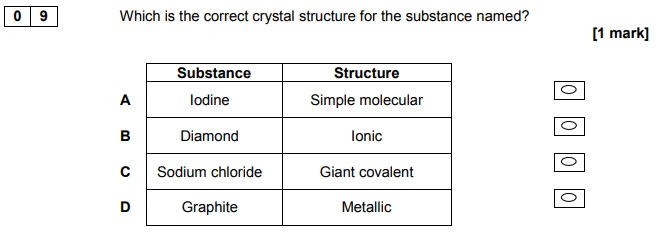ER 85.7% scored the mark as it was a straightforward question. A
 Q10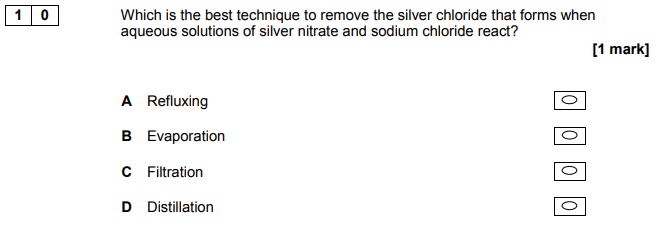ER A relatively easy practical question not needing much application of knowledge. 63.6% of students got the mark. C
 Q11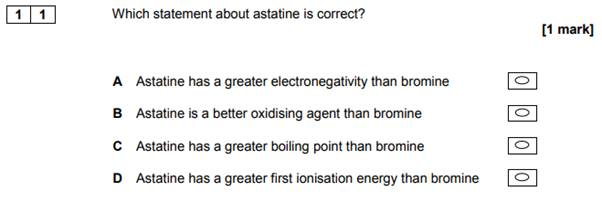ER Answered correctly by 71.7% of students highlighting this is one of the easier physical properties to remember. C
 Q12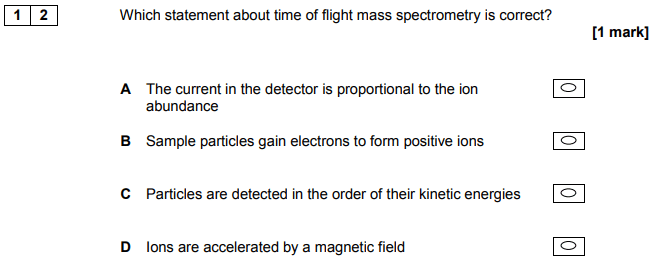ER 48.5% of students got this mark. The most common wrong answer was D, showing that some students thought that a magnetic field is involved in TOF mass spectrometry. A
 Q13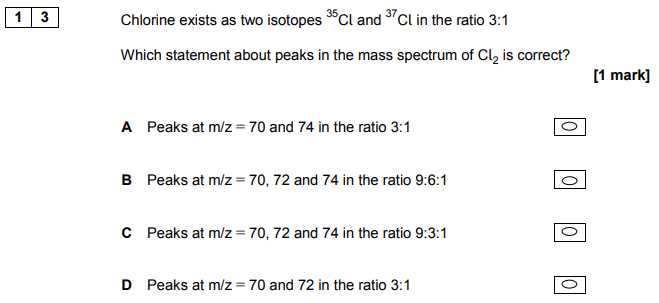ER Only 26.2% of students got this right, with the other 3 “distractors” attracting many students. This is a commonly taught example but many students could not work out the probabilities themselves. B
 Q14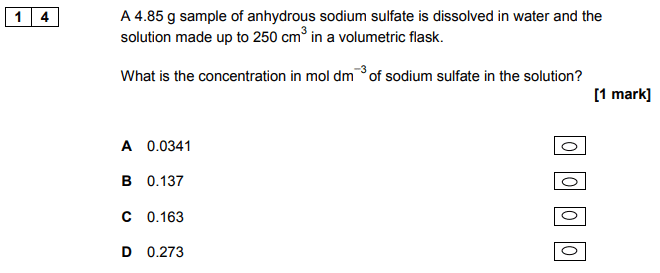ER 38.1% of students were successful. The most popular answer was C which shows that an incorrect formula (NaSO4) must have been used by these students. B
 Q15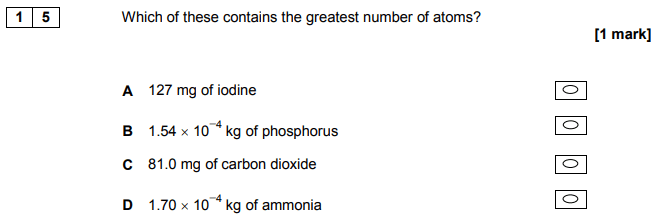ER 47.7% of students gained this mark but all other answered attracted a significant amount of students. D
 Q16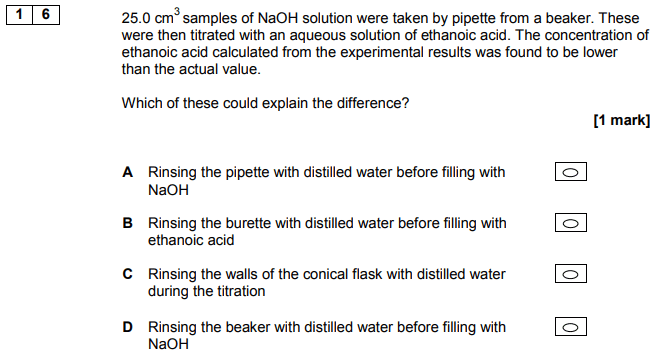ER 42.3% of students selected the correct answer. Most of the other students chose C – rinsing the walls of the conical flask with water highlighting a common misconception that is clearly not well understood. B
 Q17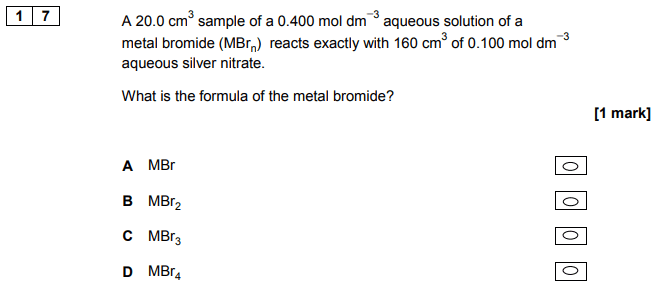ER “A surprising 66% of students got this tricky calculation right!” B
 Q18ER Although 55.5% of students got this correct, a surprising number thought that methane (A) has a 90º bond angle – it was the most popular distractor. C
 Q19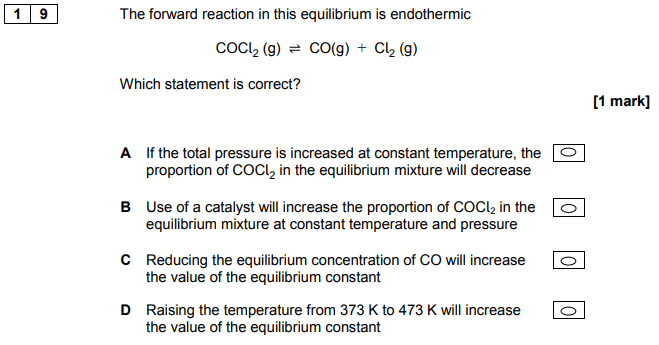ER Students showed a good understanding of qualitative equilibrium ideas, with 64% gaining this mark. D
 Q20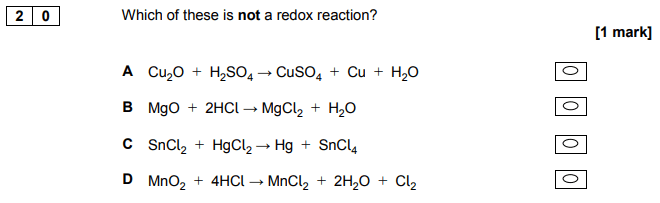ER Although 47.8% of students scored here, the three distractors all drew significant attention. B
 Q21ER 69% of students got this mark. D
 Q22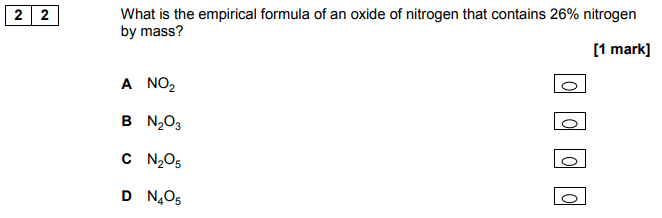ER 66.4% of students got this mark. C
 Q23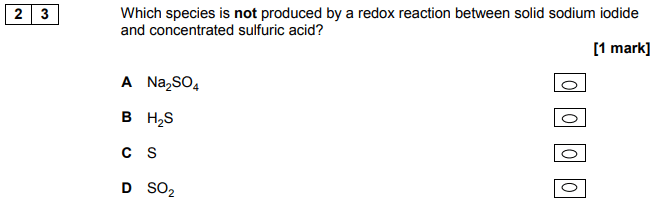ER Only 42.2% of students selected the correct answer. Perhaps many students did not appreciate that a range of sulfur-based products are formed in the reaction. A

#### If you’re a fan of studying with past papers and have your UCAT exam coming up – don’t burn out all the specimen past papers early! Our UCAT Ultimate Collection gives you a massive 6 EXTRA past papers to revise with and get the score you deserve.

GET THE BOOK FOR JUST £25